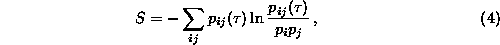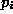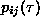Next: False nearest neighbors Up: Embedding parameters Previous: Embedding parameters

### Mutual information

The time delayed mutual information was suggested by Fraser and Swinney  as a tool to determine a reasonable delay: Unlike the autocorrelation function, the mutual information takes into account also nonlinear correlations. One has to computewhere for some partition on the real numbersis the probability to find a time series value in the i-th interval, andis the joint probability that an observation falls into the i-th interval and the observation timelater falls into the j-th. In theory this expression has no systematic dependence on the size of the partition elements and can be quite easily computed. There exist good arguments that if the time delayed mutual information exhibits a marked minimum at a certain value of, then this is a good candidate for a reasonable time delay. However, these arguments have to be modified when the embedding dimension exceeds two. Moreover, as will become transparent in the following sections, not all applications work optimally with the same delay. Our routine mutual uses Eq.(), where the number of boxes of identical size and the maximal delay time has to be supplied. The adaptive algorithm used in  is more data intensive. Since we are not really interested in absolute values of the mutual information here but rather in the first minimum, the minimal implementation given here seems to be sufficient. The related generalized mutual information of order two can be defined using the correlation sum concept (Sec., [26, 27]). Estimation of the correlation entropy is explained in Sec..Next: False nearest neighbors Up: Embedding parameters Previous: Embedding parameters

Thomas Schreiber
Wed Jan 6 15:38:27 CET 1999